Courses

# Test: Level - 2 Force And Newton’s Laws Of Motion (With Solution) - 2

## 30 Questions MCQ Test Physics Class 11 | Test: Level - 2 Force And Newton’s Laws Of Motion (With Solution) - 2

Description
This mock test of Test: Level - 2 Force And Newton’s Laws Of Motion (With Solution) - 2 for Class 11 helps you for every Class 11 entrance exam. This contains 30 Multiple Choice Questions for Class 11 Test: Level - 2 Force And Newton’s Laws Of Motion (With Solution) - 2 (mcq) to study with solutions a complete question bank. The solved questions answers in this Test: Level - 2 Force And Newton’s Laws Of Motion (With Solution) - 2 quiz give you a good mix of easy questions and tough questions. Class 11 students definitely take this Test: Level - 2 Force And Newton’s Laws Of Motion (With Solution) - 2 exercise for a better result in the exam. You can find other Test: Level - 2 Force And Newton’s Laws Of Motion (With Solution) - 2 extra questions, long questions & short questions for Class 11 on EduRev as well by searching above.
QUESTION: 1

### Concept of pseudo force is valid only in

Solution:

Pseude force is applied only for non-inertial frame.

QUESTION: 2

### A block of mass m is kept on a smooth moving wedge. If the acceleration of the wedge is equal to a, acceleration of m relative to the wedge is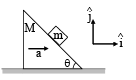Solution:

F. B. D. of block with respect to wedge.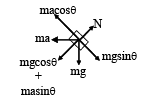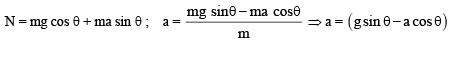QUESTION: 3

### A boy of mass 40 kg is climbing a vertical pole at a constant speed. If the coefficient of friction between his palms and the pole is 0.8 and g = 10 m/s2 the horizontal force that he is applying on the  pole is

Solution:

Here,    μ= 0. 8

Let F be horizontal force that the boy is applying on the pole.
The various forces are acting on the boy as shown in the below figure.
Frictional force.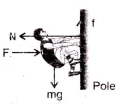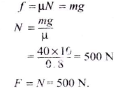QUESTION: 4

In the adjoining figure, the tension in the string connecting A and B is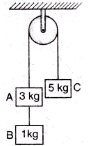Solution: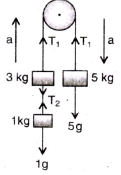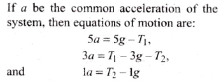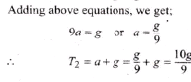QUESTION: 5

In the system shown in the adjoining figure, the acceleration of the 1 kg mass is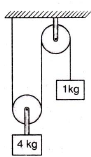Solution: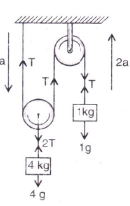Suppose a be the downward acceleration of the 4 kg mass, therefore, 2 a    is the upward acceleration of the I kg mass. Hence, equations of motion are: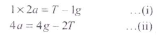Adding, after multiplying the equation (i) by 2.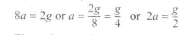Thus, the acceleration of the mass
a 1 kg is g/2 upwards.

QUESTION: 6

Two masses M1 and M2 are attached to the ends of a string which passes over a pulley attached to the top of a double inclined plane of angles of inclination α and β If M2 > M1, the acceleration ‘a’ of the system is given by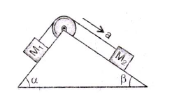Solution:

Suppose M2 moves downwards with an acceleration a and the tension in the string is T.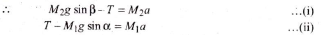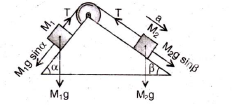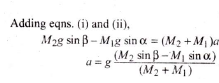QUESTION: 7

Three masses of 1 kg, 6 kg and 3 kg are connected to each other with threads and are placed on a table a as shown in the figure. What is the acceleration with which the system is moving  (Take g = 10 m/s2)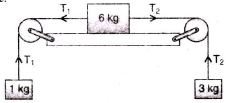Solution: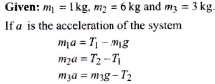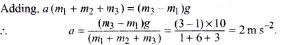QUESTION: 8

The pulley arrangements shown in figure are identical, the mass of the rope being negligible. In case I, the mass m is lifted by attaching a mass 2m to the other end of the rope. In case II, the mass m is lifted by pulling the other end of the rope with a constant downward force, F = 2 mg, where g is acceleration due to gravity. The acceleration of mass m in case I is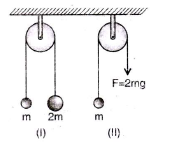Solution: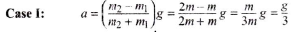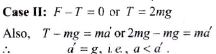QUESTION: 9

In the pulley-block arrangement shown in figure. Find the relation between acceleration of block A and B.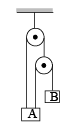Solution:

x1 + x2 = l1

Differentiating with respect to time,  we get v1 + v3 = 0

Again differentiating w.r.t. time,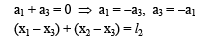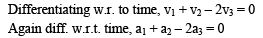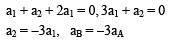QUESTION: 10

Two masses of 1 kg and 5 kg are attached to the ends of a massless string passing over a pulley of negligible weight. The pulley itself is attached to a light spring balance as shown in the figure. The masses start moving during this interval, the reading of spring balance will be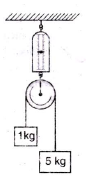Solution:

Applying the 2nd law of motion,
Equating all forces on the string carrying the 2 blocks we get T = 5g/3
The tension in spring balance = 2T
So, the reading is the mass = T/g = 10/3 Kg < 6Kg

QUESTION: 11

Two bodies of mass 4 kg and 6 kg are attached to the ends of a string passing over a pulley. A 4 kg mass is attached to the table top by another string. The tension in this string T1 is equal to (g = 10 m/s2)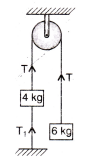Solution: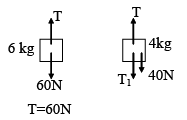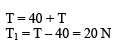QUESTION: 12

Two bodies of mass 6 kg and 4 kg are tied to a string as shown in the adjoining figure. If the table is smooth and pulley frictionless, then acceleration of mass 6 kg will be (g = 10 m/s2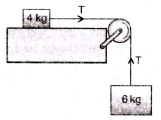Solution:
QUESTION: 13

Two masses of 8 kg and 4 kg are connected by a string as shown in the figure over a frictionless pulley. The acceleration of the system is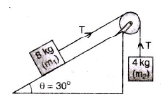Solution: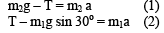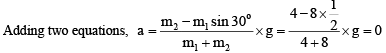QUESTION: 14

A trolley T of mass 5 kg on a horizontal smooth surface is pulled by a load of 2 kg through a uniform rope ABC of length 2 m and mass 1 kg. As the load falls from BC = 0 to BC = 2 m, its acceleration (in m/s2) changes from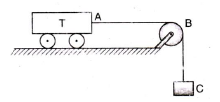Solution:

Initial force = load x g = 2 x 10 = 20 N

∴ initial acceleration = Force/Mass =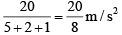Final force = (load + mass of the thread) x g = ( 2 + 1 ) x 10 = 30N

∴  Final acceleration = (30/8) m/s2

QUESTION: 15

Two wooden blocks are moving on a smooth horizontal surface such that the mass m remains stationary with respect to block of mass M as shown in figure. The magnitude of force P is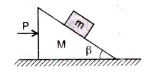Solution:

The difterent torces acting on mass m are shown in the adjoining figure. Acceleration of the system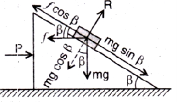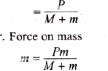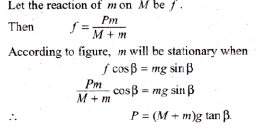QUESTION: 16

A block sliding along inclined plane as shown in figure. If the acceleration of chamber is ‘a’ as shown in the figure. The time required to cover a distance L along inclined plane is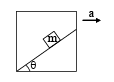Solution: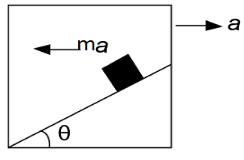Applying pseudo force ma along the left direction of the block.
The net acceleration along the incline will be aeffective​=acosθ+gsinθ
The time required will be L=0×t+½ ​aeffective​t2

⇒ √ 2L/​​ aeffective​
⇒√ 2L /acosθ+gsinθ​​

QUESTION: 17

A block of mass 4 kg is suspended through two light spring balances A and B. Then A and B will read respectively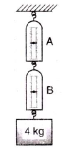Solution:

Tension is uniformly transmitted if the springs are massless.

QUESTION: 18

A light string fixed at one end to a clamp on ground passes over a fixed pulley and hangs at the other side. It makes an angle of 30o with the ground. A monkey of mass 5 kg climbs up the rope. The clamp can tolerate a vertical force of 40 N only. The maximum acceleration in upward direction with which the monkey can climb safely is (neglect friction and take g = 10 m/s2)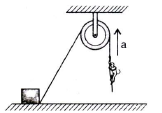Solution:

Let T be the tension in the string.

The upward force exerted on the clamp = T sin 30o = T/2

Given: (T/2) = 40 N   or   T = 80 N

If ‘a’ is the acceleration of the monkey in upward direction,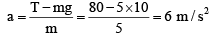QUESTION: 19

If the normal reactional force is doubled, the coefficient of friction is

Solution:

Coefficient of static friction does not depend upon normal reaction.

QUESTION: 20

The force acting on the block pushes it, then pushing of the block will be possible along the surface, if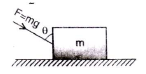Solution:

In this case, vertical component of the force increases the normal reaction, i.e.,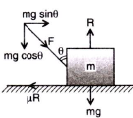R = mg + mg cos θ = mg(1 + cosθ)

Hence, block can be pushed along the horizontal surface when Horizontal component of force > frictional force,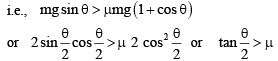QUESTION: 21

An object is placed on the surface of a smooth inclined plane of inclination θ It takes time ‘t’ to reach he bottom. If the same object is allowed to slide down a rough inclined plane of inclination θ it takes time nt to reach the bottom where n is a number greater than 1. The coefficient of friction μ  is given by

Solution:

On smooth inclined plane:
Acceleration of the body = g sin θ If s be the distance travelled, then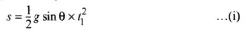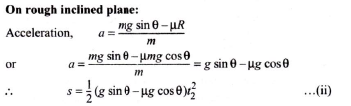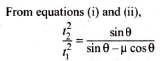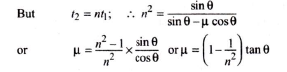QUESTION: 22

The frictional force between two surfaces is independent of

Solution:

The frictional force between two surfaces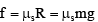where μs depends on the nature of surface and their materials. Thus, frictional force is independent of area of contact of the surfaces.

QUESTION: 23

A body of mass 2 kg rests on a rough inclined plane making an angle 30o with the horizontal. The coefficient of static friction between the block and the plane is 0.7. The frictional force on the block is

Solution:

The body is at rest on the inclined plane, as μ > tan θ  force of friction = mg sin 30o = 2 x 9.8 x (1/2) = 9.8 N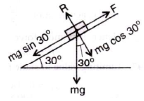QUESTION: 24

A man walks over a rough surface, the angle between the force of friction and the instantaneous velocity of the person is

Solution:

When a man walks on a rough surface, it is the frictional force which is responsible for motion, i.e, required angle between frictional force and instantaneous velocity is zero.

QUESTION: 25

A heavy block of mass M is slowly placed on a conveyor belt moving with a speed v. The coefficient of friction between the block and the belt is μ. Through what distance will the block slide on the belt?

Solution:

Frictional force on the block = μMg

It will be causing acceleration a =μMg/M = μg

The block slide on the ball till its velocity becomes ‘v’ given by the equation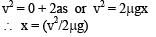QUESTION: 26

A uniform rope of length l lies on a table. If the coefficient of friction is m, then the maximum length l1of the part of this rope which can overhang from the edge of the table without sliding down is

Solution: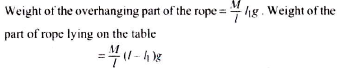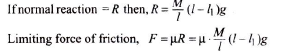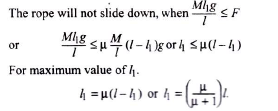QUESTION: 27

A body is moving with uniform velocity of 2 m/s on a rough level surface. The frictional force on it is 10 N. If the body moves with velocity 4 m/s, the force of friction will be

Solution:

Kinetic friction is constant, hence frictional force will remain same (= 10 N).

QUESTION: 28

The coefficient of static friction μ  between block A of mass 2kg and the table as shown in the figure is 0.2. What would be the maximum mass value of block B so that the two blocks do not move? The string and the pulley are assumed to be smooth and massless (g = 10 m/s2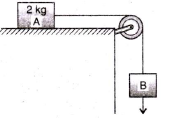Solution:

For the equilibrium,  mBg = μmAg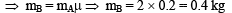QUESTION: 29

At any instant if the velocity of point A is 10 m/s then calculate the velocity of point B?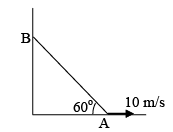Solution: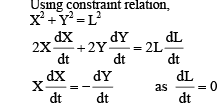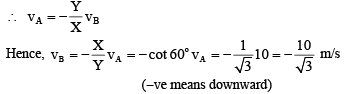QUESTION: 30

Frictional force acting on the block is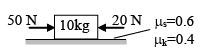Solution:

Net force = 50 - 20 = 30 N
Limiting friction on the body = u (static) × m × g = 0.6 × 10 × 10 = 60 N.
F = 30 N is less than the limiting friction so the body is static. So, a = 0.
Force of friction acting on the body is static friction, f = driving force = 30 N.